# The Ideal Diode

The following article explains the theory of operation of an ideal diode circuit implemented using a p-channel MOSFET and a matched PNP transistor pair. Typical applications for the ideal diode are devices such as solar chargers, where power efficiency is of a great importance.

## Introduction

Diodes are devices that allow the electric current to flow in only one direction. As shown in the image below, the current is allowed to flow from the anode towards the cathode but not the other way around.

Diodes have many applications ranging from simple reverse polarity protection to full bridge rectifiers. There is plenty of available material explaining the diode basics, therefore I would like to skip this part and only cover one particular aspect of diodes which makes them rather power inefficient devices. This article shall cover the forward voltage drop denoted in the datasheet as.is the voltage measured between the anode and the cathode of a diode subjected to an electric current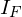in its forward direction. Whereas the anode corresponds to the positive side and the cathode to the negative. Typical values ofare 0.6V for a standard silicone Diode and as low as 0.1V for a Schottky type diode. The value ofis a function of the forward bias currentas shown in the diagram below.

The above diagram plots the forward voltage drop(horizontal axis) versus the forward bias current(vertical axis) for a 1N4007 or similar diode. As one can see,can go as high as 1 Volt for 1 Ampere of current, which results in a dissipated power loss of 1 Watt.

As the name of this article suggests, the ideal diode is one which exhibits no (or very little) power loss. Thus, it should have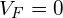(or close to) for a wide range of. Presented in this article is a small circuit that mimics the behavior of of a diode with a near zero forward voltage drop.

## Circuit Diagram

As seen in the schematic below, the ideal diode consists of a p-channel MOSFET Q2 and a voltage comparator consisting of a matched PNP transistor pair Q1A and Q1B.

## MOSFET

The IRLML2244 p-channel MOSFET Q2 is driven in the reverse direction, whereas its drain pin 3 is connected to the input voltageand its source pin 2 provides the output voltage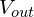. This ensures that the MOSFET’s intrinsic diode is aligned in the direction of forward current flow and prevents any reverse current from flowing through it.

This MOSFET exhibits a very low static drain-to-source on-resistance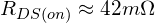at a gate-source voltage of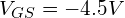and a drain current of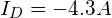(see datasheet). The resulting measured forward voltage drop amounts to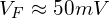at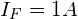.

## Voltage Comparator

A voltage comparator circuit has been implemented around the PNP transistors Q1A and Q1B. It is important that these transistors have identical characteristics, otherwise the comparator will not have the required precision. Thus, these transistors part of a BC857BS matched transistor pair sharing the same package. Having both transistors inside one physical package ensures that they are thermally coupled and avoids diverging characteristics due to different junction temperatures.

The voltage comparator compares the voltagestoand controls the MOSFET gate voltage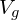across the resistor R2.

The following equations apply for the voltage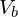between the transistor’s base and the power supply ground: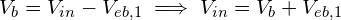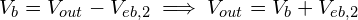where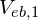and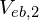are the emitter-base voltages of transistors Q1A and Q1B. And: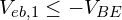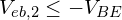Where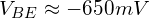is the specified base-emitter voltage drop for BC857BS (see datasheet). Due to the properties of the base-emitter junction which is essentially a diode, the voltagesandare clamped to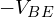. A current can only flow through the emitter-base junction of Q1A or Q1B if the corresponding emitter-base voltageorreaches (gets slightly higher than).

## Forward Bias

The following holds true when the ideal diode is forward biased: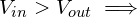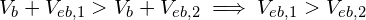The larger ofandwill be clamped towhich leads to the following statements: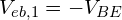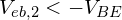Consequently, current will flow through the emitter-collector path of transistor Q1A while no current will flow through the emitter-collector path of Q1B. Thus, the voltageacross the resistor R2 will be equal (or near equal) to 0V. This will lead to a negative gate-source voltage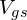and cause the MOSFET Q1B to turn on.

## Reverse Bias

The following holds true when the ideal diode is reverse biased: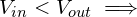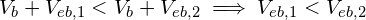The larger ofandwill be clamped towhich leads to the following statements: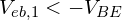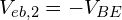Consequently, current will flow through the emitter-collector path of transistor Q1B while no current will flow through the emitter-collector path of Q1A. Thus, the voltageacross the resistor R2 will be equal (or near equal) to. This will lead to near zero gate-source voltageand cause the MOSFET Q1B to turn off.

## PCB Layout

The circuit has been implemented on a SMD prototyping board as shown in the pictures below. The surface mount MOSFET, transistor pair and 0805 resistors have been connected using jumper wires. The three terminals of the ideal diode have been connected to a pin header.

The left picture shows the top side of the PCB with the visible MOSFET (3 pin package) and dual transistor (6 pin package). The right picture shows the backside of the PCB with the two 0805 resistors. Note that the pads on both sides are connected through the holes.

Following are the pin assignments on the pin header, assuming pin 1 is the leftmost pin and pin 3 is the rightmost pin on the left picture:

• Pin 1:• Pin 2: GND
• Pin 3:## Bill of Material

Following is the list of parts required for building the ideal diode. Please consider supporting this website by purchasing your the required parts using the affiliate links below: